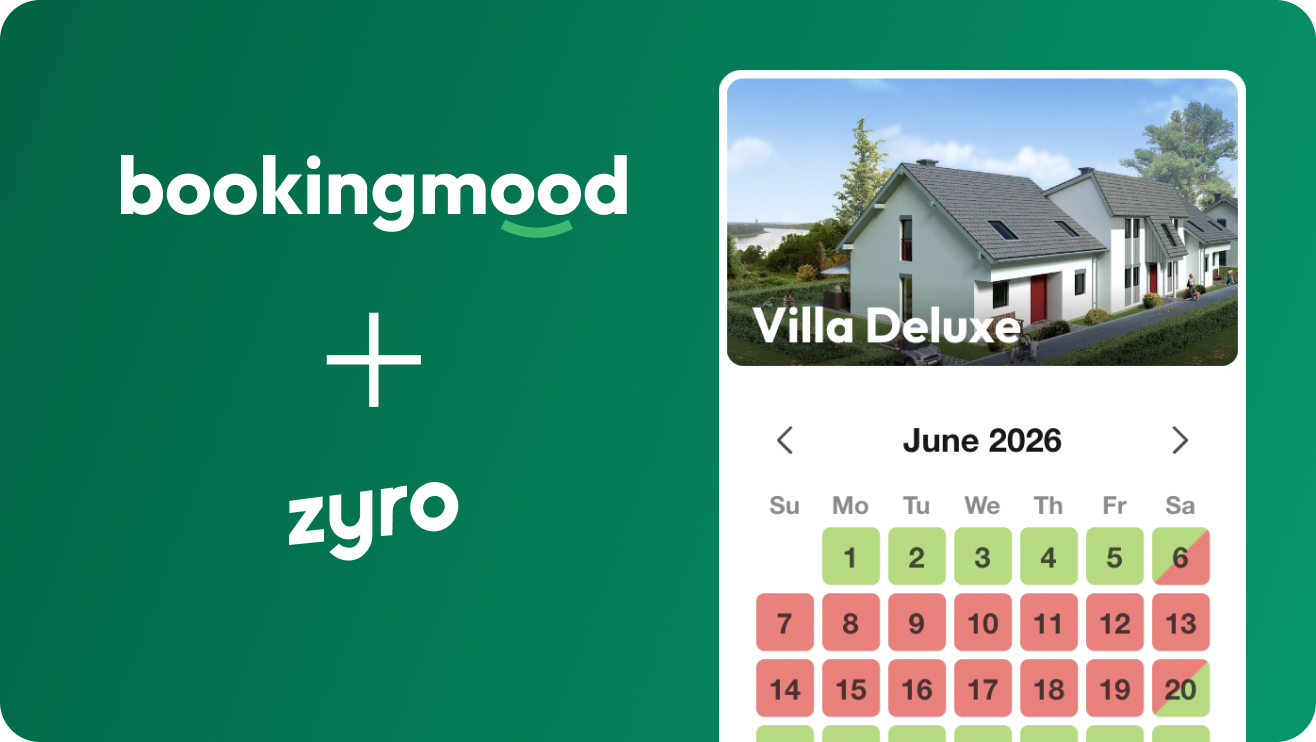# Booking calendar for Zyro## Need a booking calendar for Zyro? Try Bookingmood!self.__wrap_n=self.__wrap_n||(self.CSS&&CSS.supports("text-wrap","balance")?1:2);self.__wrap_b=(e,t,n)=>{let r=(n=n||document.querySelector(`[data-br="\${e}"]`)).parentElement,i=e=>n.style.maxWidth=e+"px";n.style.maxWidth="";let o=r.clientWidth,a=r.clientHeight,l=o/2-.25,s=o+.5,c;if(o){for(i(l),l=Math.max(n.scrollWidth,l);l+1<s;)i(c=Math.round((l+s)/2)),r.clientHeight===a?s=c:l=c;i(s*t+o*(1-t))}n.__wrap_o||"undefined"!=typeof ResizeObserver&&(n.__wrap_o=new ResizeObserver(()=>{self.__wrap_b(0,+n.dataset.brr,n)})).observe(r)};self.__wrap_n!=1&&self.__wrap_b(":R5aqpamlla:",1)

Bookingmood is a booking calendar that's 100% compatible with Zyro. In just a few minutes you'll turn your Zyro website into a booking channel.

View the Zyro manual